# ISEE Middle Level Math : Fractions

## Example Questions

### Example Question #11 : How To Find The Decimal Equivalent Of A Fraction

Write as a decimal: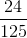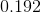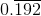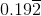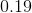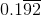Explanation:

Divide 24 by 125: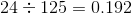### Example Question #12 : Fractions

What is the decimal equivalent to this fraction?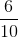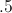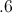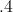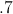Explanation:

Divide: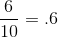### Example Question #13 : Fractions

What is the equivalent decimal representation?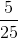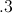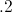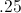Explanation:

Divide: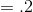### Example Question #14 : Fractions

What is the equivalent decimal representation?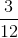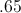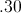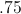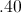Explanation:

Divide: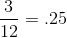### Example Question #15 : Fractions

Which answer choice is equivalent to this fraction?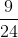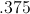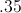Explanation:

Divide: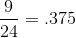### Example Question #16 : Fractions

What is the equivalent decimal representation?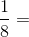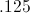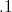Explanation:

Divide: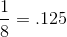### Example Question #17 : Fractions

Which decimal is equivalent to this fraction?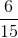Explanation:

Divide: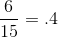### Example Question #18 : Fractions

Which is equivalent to this fraction?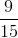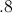Explanation:

Divide: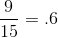### Example Question #11 : Fractions

Write 4.8 as an improper fraction in lowest terms.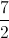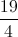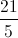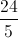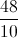Explanation:

This is forty-eight tenths; write this as a fraction, then simplify: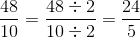### Example Question #11 : Fractions

Which answer choice is equivalent to 60%?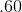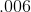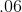Explanation:

Find the decimal equivalent of 60%: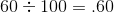### All ISEE Middle Level Math Resources